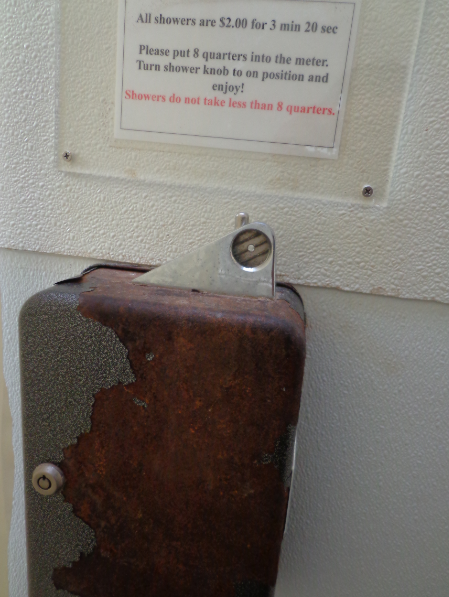# Sunday September 10th

## Good news first…

• I just got back in to find that I’ve been accepted to LinkedIn’s recruitment for students who are about to look for work. I was invited to apply by two of LinkedIn’s recruiters. So excited for the event! :)

## I got back in from Catalina.

• It was my first time sailing over, and my first time there.

• We left at noon on Friday, and got back by 5pm on Sunday.

## Water is a huge problem on the island…

• You put in two dollars in quarters for three minutes and 20 seconds of water for a shower. I actually thought it was about 5 minutes..way more than I needed!## Saturday view

• We took a dinghy over to the camp ground and had a BBQ with some people from other marinas. Also, the night before, there was a dinghy roundup where we passed around food!## Our neighbour!

• He’s also from MDR..They had a sweet BBQ on board.

• Boats here are on a mooring. Ours was L16 (Lima 16)## View from Dinghy passing by boats## View of Kayaks from Land…## Really good answer to a question on Quora…

• How to become an expert. Basically this answer suggested that the person enquiring take a library in a language and rewrite it, and compare it with the original. Really great answer.

## Kata

• return Two’s complement ie. switch ones with zeros
``````function onesComplement(n) {
var arr = []
for (var i = 0; i < n.length; i++){
if (n[i] == '1'){
arr.push('0')
}
else if (n[i] == '0'){
arr.push('1')
}
}
g = arr.join('')

return g
};
``````
• Find the value where 3^k < N, given N
``````# there is nothing to explain
# this is english -> mathematics
def largestPower(N):
import math
num = 0
while (math.pow(3, num) < N):
num = num + 1
return num - 1
``````
• multiply values in string and return the answer as a string
``````function arrMultiply(arr){
var total = 1
for (var i = 0; i < arr.length; i++){
// set total to 0 if any value is 0
if (arr[i] == '0'){
total = 0
}
else
{
total = total * parseInt(arr[i])
}
}
return(total.toString())
}
``````
• Insert a dash between any two odd numbers.
``````function insertDash(num) {
var arr = []
var a = num.toString()

// check for both
for (var i = 0; i < a.length; i++){
if ((a[i] % 2 !== 0) && (a[i+1] % 2 !== 0))
arr.push(a[i]+'-')
else{
arr.push(a[i])
}
}
var b = arr.join('')

// strip off any dashes at end
if (b[b.length-1] == '-')
return(b.slice(0,b.length-1))
else{
return(b)
}
}
``````
• sum of primes up to number given
``````def summationOfPrimes(primes):
total = 0
for num in range(1,primes + 1):
if num > 1:
for i in range(2,num):
if (num % i) == 0:
break
else:
total = total + num